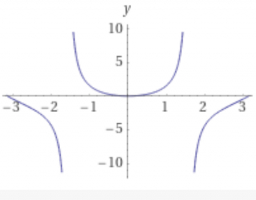# Function x*tanx

Functions:

f(x)=xtanx
f(x)=(e^x)/((e^x)+1)

Find;
i)vertical and horizontal assyptotes
iii)the interval of decrease and increase
iii)Local maxima and local minima
iv)interval of concavity and inflection.
And sketch the graph.

min =  0

### Step-by-step explanation:Did you find an error or inaccuracy? Feel free to write us. Thank you!

Tips for related online calculators
Need help calculating sum, simplifying, or multiplying fractions? Try our fraction calculator.